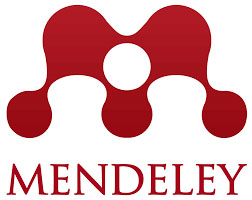rscosan.com

# Paper: Generalizations of generating functions for basic hypergeometric orthogonal polynomials

 PSR PRESS (Pakistan) | ISSN: 2616-4906 | Impact Factor: n/a title## Generalizations of generating functions for basic hypergeometric orthogonal polynomials

Cohl, H. S., Costas-Santos, R. S., Hwang, P. R. and Wakhare, T. V. Open Journal of Mathematical Sciences 6 no. 1 (2022), 248– 261

## Abstract

We derive generalized generating functions for basic hypergeometric orthogonal polynomials by applying connection relations with one free parameter to them. In particular, we generalize generating functions for the Askey-Wilson, continuous $$q$$-ultraspherical/Rogers, little $$q$$-Laguerre/Wall, and $$q$$-Laguerre polynomials. Depending on what type of orthogonality these polynomials satisfy, we derive corresponding definite integrals, infinite series, bilateral infinite series, and $$q$$-integrals.

369 KB Preprint (PDF, 16 pages)

## BibTeX

@article {cohlcostashwangwakhare2022,
AUTHOR={Cohl, H. S.; Costas-Santos, R. S.; Hwang, P. R.  and Wakhare, T. V.},
TITLE={Generalizations of generating functions for basic hypergeometric orthogonal polynomials},
FJOURNAL={Open Journal of Mathematical Sciences},
VOLUME={6},
YEAR={2022},
NUMBER={1},
PAGES={248--261},
ISSN={2523-0212},
MSC={33D45; 05A15; 33D15; 33C45; 33C20; 34L10; 30E20}
DOI={10.30538/oms2022.0190},
URL={http://doi.org/10.30538/oms2022.0190},
}